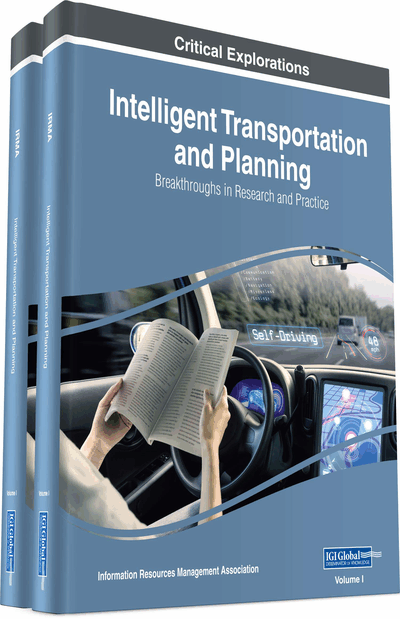# On Solving Multiobjective Transportation Problems With Fuzzy Random Supply and Demand Using Fuzzy Goal Programming

Animesh Biswas (University of Kalyani, Kalyani, India) and Nilkanta Modak (University of Kalyani, Kalyani, India)
DOI: 10.4018/978-1-5225-5210-9.ch035
Available
\$33.75
List Price: \$37.50
10% Discount:-\$3.75
TOTAL SAVINGS: \$3.75

## Abstract

In this article a fuzzy goal programming model is developed to solve multiobjective unbalanced transportation problems with fuzzy random parameters. In model formulation process the cost coefficients of the objectives are considered as fuzzy numbers and the supplies and demands are considered as fuzzy random variables with known fuzzy probability distribution from the view point of probabilistic as well as possibilistic uncertainties involved with the model. A fuzzy programming model is first constructed by applying chance constrained programming methodology in fuzzy environment. Then, the model is decomposed on the basis of the tolerance ranges of the fuzzy numbers associated with it. The individual optimal solution of each decomposed objectives is found in isolation to construct the membership goals of the objectives. Finally, priority based fuzzy goal programming technique is used to achieve the highest degree of each of the defined membership goals to the extent possible by minimizing the under deviational variables and thereby obtaining optimal allocation of products by using distance function in a cost minimizing decision making environment. An illustrative example is solved and compared with existing technique to explore the potentiality of the proposed methodology.
Chapter Preview
Top

## Introduction

In today’s highly competitive market the organization have a challenge to find better ways to create and deliver products to the customers. When and how much of products is to be sent to the customers in a cost-effective manner is even more challenging. Transportation model is a powerful frame work to overcome this situation. The physical distribution of products from supply to demand points, minimization of the cost and time, requirement of goods at each demand point, variety of roots and manners, are the basic point of a transportation problem (TP). The basic transportation model was originated by Hitchcock (1941) and discussed in details by Koopmans (1949).

TP is considered as a special type of multiobjective programming problems (MOPPs) which arises in many practical applications. Multiobjective transportation problem (MOTP) is a special class of multiobjective linear programming problem with non-commensurable and conflicting objectives as well as constraints with special structure. Most of the researchers have given serious attention on solving MOTPs in recent times. In conventional TPs, it is assumed that decision makers (DMs) are sure about the precise value of transportation costs, availability and demand of the products. But, in real world applications all these parameters are not known precisely due to some uncontrollable factors. The probability theory and fuzzy set theory are the avenues to analyze these uncertain situations.

To deal with the parameters involving random variables associated with the system constraints Charnes and Cooper (1959) introduced chance constrained programming (CCP) technique for solving probabilistic linear programming problems. Generalizing the concept of CCP methodology Dantzig (1963) formulated stochastic programming. The method is further studied by Vajda (1972), Sengupta (1972), Luhandjula (1983). Considering the random variables associated with supply and demand Ringuest and Rinks (1987) proposed an interactive algorithm for multiobjective stochastic TP. Azadi & Saen, (2013) developed a chance constrained programming model for selecting third party reverse logistics providers.

It is to be noted here that in a TP, the cost of transporting unit products or time required for transporting unit product may not be defined precisely due to different uncontrollable factors. Realizing the above fact Oheigeartaigh (1982) proposed an algorithm for solving TPs where the capacities and requirement are fuzzy sets (Zadeh, 1965) with linear or triangular membership functions. Chanas et al. (1984) presented fuzzy linear programming model (Zimmermann, 1978) for solving TP with crisp cost coefficients and fuzzy supply and demand values. The TP with fuzzy cost coefficients expressed as fuzzy numbers was studied by Chanas and Kuchta (1996). Liu & Kao (2004) described a method for solving fuzzy TP based on extension principle. A two-stage cost minimizing fuzzy TP with supply and demand as trapezoidal fuzzy numbers presented by Gani and Razak (2006). Li et al. (2008) proposed a new method based on goal programming for solving fuzzy TP with fuzzy costs. Lin (2009) applied genetic algorithm for solving TP with fuzzy coefficients. Ghatee, Hashemi, Zarepisheh and Khorram (2009) introduced a method for solving fuzzy minimal cost flow problem with fuzzy costs. A technique for solving fully fuzzy transportation problem was presented by Kumar and Kaur (2011). Roy and Mahapatra (2014) presented a methodology for solving solid transportation problem with stochastic supply and demands. Radhakrishnan and Anukokila (2014) presented a methodology for fractional solid TP with interval cost using fractional goal programming approach. Erol and Yilmaz (2016) presented a literature survey for hazardous materials transportation.

## Complete Chapter List

Search this Book:
Reset